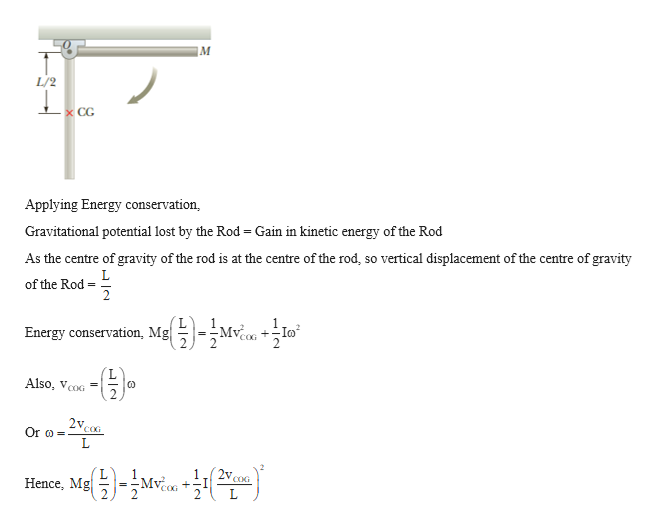Question
1 views

A uniform thin rod of length L and mass M is free
to rotate on a frictionless pin passing through one end
(Fig. P8.47). The rod is released from rest in the horizontal
position. (a) What is the speed of its center of gravity when
the rod reaches its lowest position? (b) What is the tangential
speed of the lowest point on the rod when it is in the
vertical position?

check_circle

Step 1

Given:

Length of rod  = L

Mass of rod = M

Step 2

Calculating the speed of its centre of gravity when the rod reaches its lowest position:help_outlineImage Transcriptioncloseм L/2 x CG Applying Energy conservation, Gravitational potential lost by the Rod = Gain in etic energy of the Rod As the centre of gravity of the rod is at the centre of the rod, so vertical displacement of the centre of gravity of the Rod = = 1. Energy conservation, Mg-Mvền +lo +-Io? Also, VcoG %3D 2vcoG Or o = COG Hence, Mg fullscreen
Step 3

Calculating the speed of its centre of gravit...help_outlineImage TranscriptioncloseML? Substituting moment of inertia of rod about the pivot point, I=- 3 Solving for speed of centre of gravity, 1( ML? 2v, Mg-Mvền. COG COG ME-Mv.o Mv 3 2 COG COG 2 Mg COG 3gL VCOG fullscreen

### Want to see the full answer?

See Solution

#### Want to see this answer and more?

Solutions are written by subject experts who are available 24/7. Questions are typically answered within 1 hour.*

See Solution
*Response times may vary by subject and question.
Tagged in

### Angular Motion